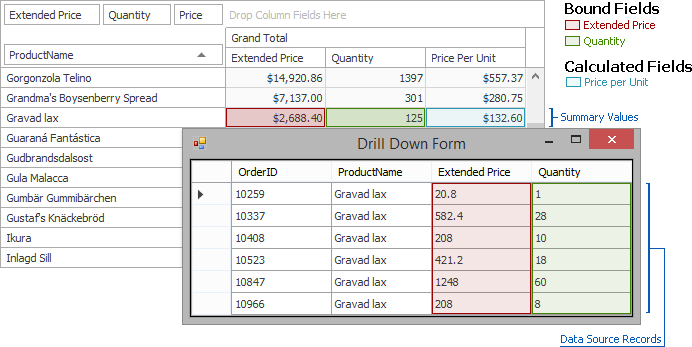# PivotGridField.UnboundExpressionMode Property

Gets or sets how the unbound expression for the specified data field is calculated.

Namespace: DevExpress.Xpf.PivotGrid

Assembly: DevExpress.Xpf.PivotGrid.v20.2.dll

## Declaration

``public FieldUnboundExpressionMode UnboundExpressionMode { get; set; }``

## Property Value

Type Description
FieldUnboundExpressionMode

An FieldUnboundExpressionMode object that specifies how the unbound expression for the specified data field is calculated.

Available values:

Name Description
Default

The default parameter value.

UseSummaryValues

An unbound expression is calculated against summary values.

DataSource

An unbound expression is calculated against each data source record, and the resulting values are summarized.

UseAggregateFunctions

An unbound expression is calculated using aggregates.

## Remarks

Unbound expressions can be calculated against summary values, or values stored in the underlying data source.

The image below shows the PivotGrid control with the drill down form invoked. The ‘Price Per Unit’ column is unbound, and the expression is specified as [Extended Price] / [Quantity].• If the ‘Price Per Unit’ field’s UnboundExpressionMode property is set to FieldUnboundExpressionMode.DataSource, the unbound expression is calculated against each data source record, and then the resulting values are summarized. In the pivot grid shown above, the ‘Price Per Unit’ column value in the ‘Gravad lax’ row will be calculated as follows:

Sum([Extended Price] / [Quantity]) = 20.8 / 1 + 582.4 / 28 + 208 / 10 + 421.2 / 18 + 1248 / 60 + 208 / 8 = 132.6

• If the field’s UnboundExpressionMode property is set to UnboundExpressionMode.UseSummaryValues, the expression is calculated against summary values, as follows:

Sum([Extended Price]) / Sum([Quantity]) = (20.8 + 582.4 + 208 + 421.2 + 1248 + 208) / (1 + 28 + 10 + 18 + 60 + 8) = 2688.4 / 125 = 21.5

• If the field’s UnboundExpressionMode property is set to FieldUnboundExpressionMode.UseAggregateFunctions, an unbound expression is calculated using aggregates.

Note

You can refer to the field using the PivotGridField.ExpressionFieldName property, which automatically returns the required identifier based on the currently enabled mode.

Note

Unbound expressions evaluated against hidden fields and fields located in the Filter Area return the ‘Error’ value if the UnboundExpressionMode property is set to UnboundExpressionMode.UseSummaryValues, because summary values for these fields are not calculated.